# Mod2 3. Acid Base Equilibria.pptx

4 de Apr de 2023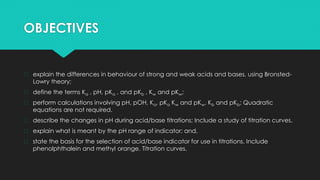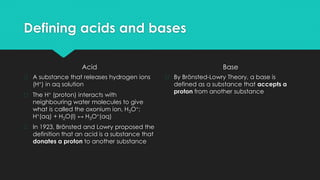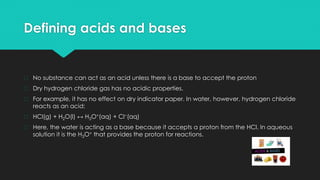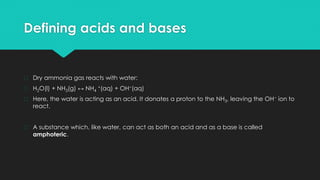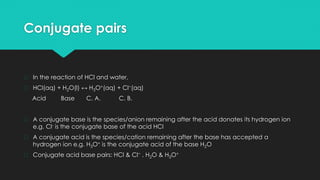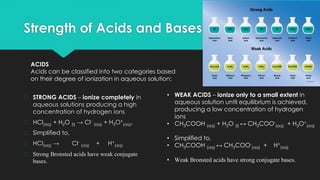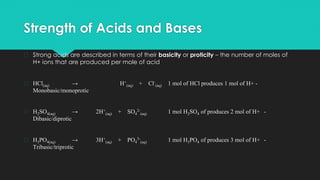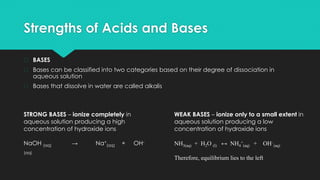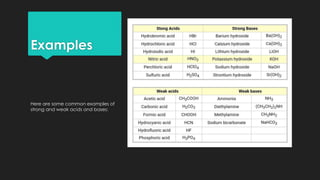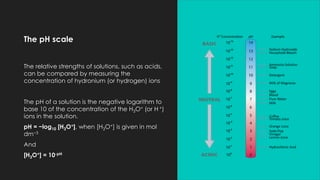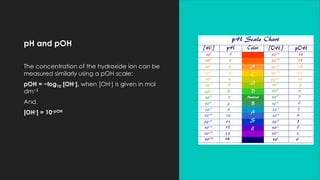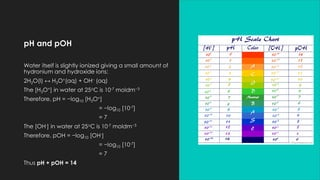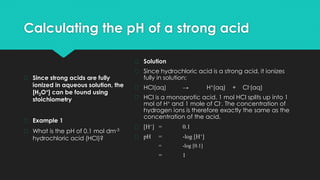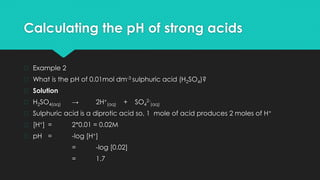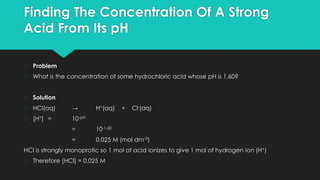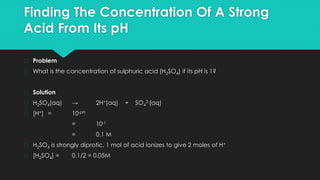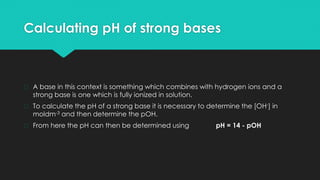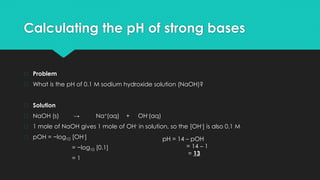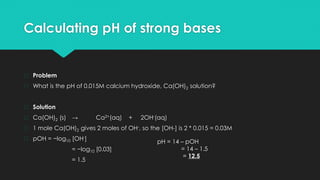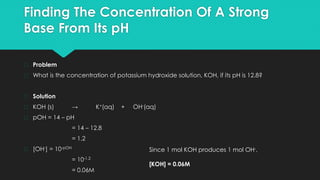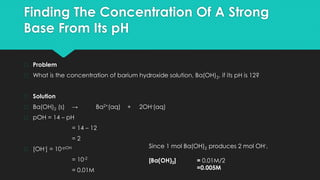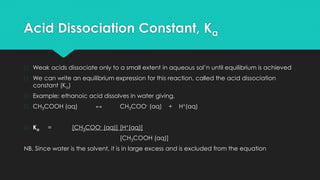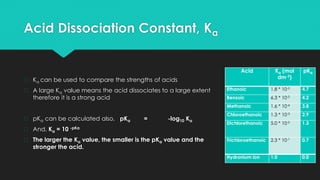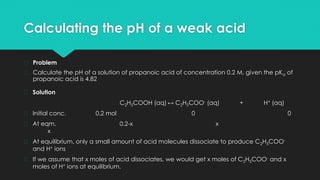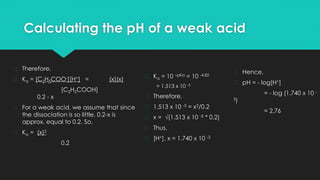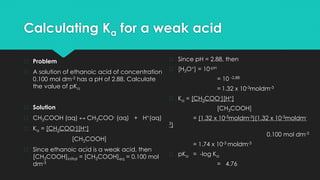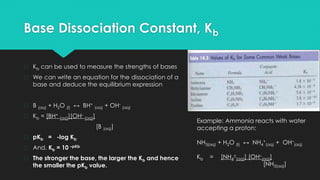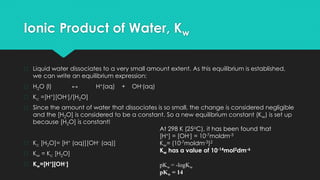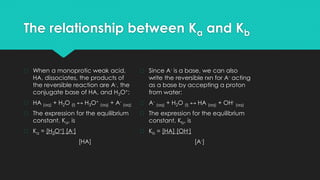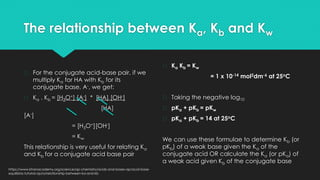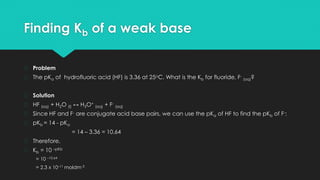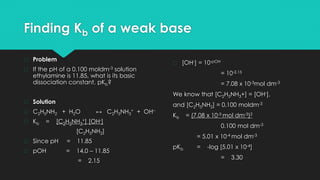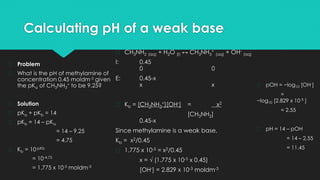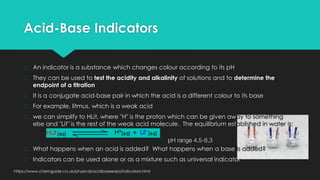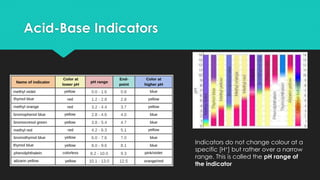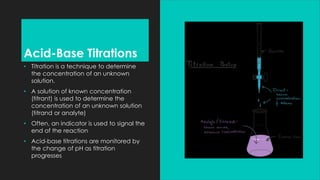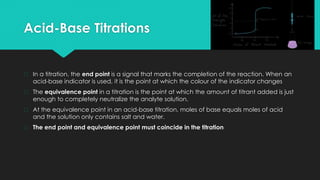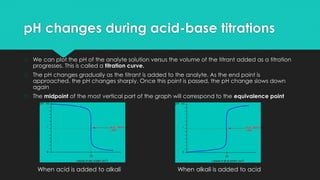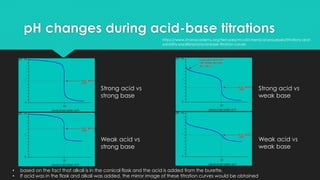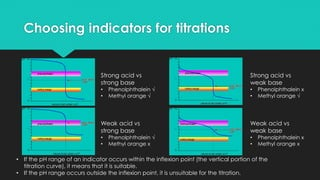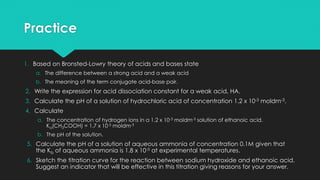1 de 45

### Mod2 3. Acid Base Equilibria.pptx

• 1. MOD 2. 3. ACID BASE EQUILIBRIA
• 2. OBJECTIVES � explain the differences in behaviour of strong and weak acids and bases, using Bronsted- Lowry theory; � define the terms Ka , pH, pKa , and pKb , Kw and pKw; � perform calculations involving pH, pOH, Ka, pKa Kw and pKw, Kb and pKb; Quadratic equations are not required. � describe the changes in pH during acid/base titrations; Include a study of titration curves. � explain what is meant by the pH range of indicator; and, � state the basis for the selection of acid/base indicator for use in titrations. Include phenolphthalein and methyl orange. Titration curves.
• 3. Defining acids and bases Acid � A substance that releases hydrogen ions (H+) in aq solution � The H+ (proton) interacts with neighbouring water molecules to give what is called the oxonium ion, H3O+: H+(aq) + H2O(l) ↔ H3O+(aq) � In 1923, Brönsted and Lowry proposed the definition that an acid is a substance that donates a proton to another substance Base � By Brönsted-Lowry Theory, a base is defined as a substance that accepts a proton from another substance
• 4. Defining acids and bases � No substance can act as an acid unless there is a base to accept the proton � Dry hydrogen chloride gas has no acidic properties. � For example, it has no effect on dry indicator paper. In water, however, hydrogen chloride reacts as an acid: � HCl(g) + H2O(l) ↔ H3O+(aq) + Cl−(aq) � Here, the water is acting as a base because it accepts a proton from the HCl. In aqueous solution it is the H3O+ that provides the proton for reactions.
• 5. Defining acids and bases � Dry ammonia gas reacts with water: � H2O(l) + NH3(g) ↔ NH4 +(aq) + OH−(aq) � Here, the water is acting as an acid. It donates a proton to the NH3, leaving the OH− ion to react. � A substance which, like water, can act as both an acid and as a base is called amphoteric.
• 6. Conjugate pairs � In the reaction of HCl and water, � HCl(aq) + H2O(l) ↔ H3O+(aq) + Cl−(aq) Acid Base C. A. C. B. � A conjugate base is the species/anion remaining after the acid donates its hydrogen ion e.g. Cl- is the conjugate base of the acid HCl � A conjugate acid is the species/cation remaining after the base has accepted a hydrogen ion e.g. H3O+ is the conjugate acid of the base H2O � Conjugate acid base pairs: HCl & Cl− , H2O & H3O+
• 7. Conjugate Pairs � Identify the conjugate acid base pairs in the following equations: � H2O (l) + NH3 (aq) ↔ NH4 + (aq) + OH- (aq) � HCl(aq) + NH3 (l) ↔ NH4 + (aq) + Cl- (aq) � HSO4 - (aq) + OH- (aq) ↔ SO4 2- (aq) + H2O (l) � H3O+ (aq) + OH- (aq) ↔ 2 H2O (l)
• 8. Strength of Acids and Bases � STRONG ACIDS – ionize completely in aqueous solutions producing a high concentration of hydrogen ions � HCl(aq) + H2O (l) → Cl- (aq) + H3O+ (aq), � Simplified to, � HCl(aq) → Cl- (aq) + H+ (aq) � Strong Bronsted acids have weak conjugate bases. • WEAK ACIDS – ionize only to a small extent in aqueous solution until equilibrium is achieved, producing a low concentration of hydrogen ions • CH3COOH (aq) + H2O (l) ↔ CH3COO- (aq) + H3O+ (aq) • Simplified to, • CH3COOH (aq) ↔ CH3COO- (aq) + H+ (aq) • Weak Bronsted acids have strong conjugate bases. ACIDS Acids can be classified into two categories based on their degree of ionization in aqueous solution:
• 9. Strength of Acids and Bases � Strong acids are described in terms of their basicity or proticity – the number of moles of H+ ions that are produced per mole of acid � HCl(aq) → H+ (aq) + Cl- (aq) 1 mol of HCl produces 1 mol of H+ - Monobasic/monoprotic � H2SO4(aq) → 2H+ (aq) + SO4 2- (aq) 1 mol H2SO4 of produces 2 mol of H+ - Dibasic/diprotic � H3PO4(aq) → 3H+ (aq) + PO4 3- (aq) 1 mol H3PO4 of produces 3 mol of H+ - Tribasic/triprotic
• 10. Strengths of Acids and Bases � BASES � Bases can be classified into two categories based on their degree of dissociation in aqueous solution � Bases that dissolve in water are called alkalis STRONG BASES – ionize completely in aqueous solution producing a high concentration of hydroxide ions NaOH (aq) → Na+ (aq) + OH- (aq) WEAK BASES – ionize only to a small extent in aqueous solution producing a low concentration of hydroxide ions NH3(aq) + H2O (l) ↔ NH4 + (aq) + OH– (aq) Therefore, equilibrium lies to the left
• 11. Examples Here are some common examples of strong and weak acids and bases:
• 12. The pH scale The relative strengths of solutions, such as acids, can be compared by measuring the concentration of hydronium (or hydrogen) ions The pH of a solution is the negative logarithm to base 10 of the concentration of the H3O+ (or H +) ions in the solution. pH = −log10 [H3O+], when [H3O+] is given in mol dm−3 And [H3O+] = 10-pH
• 13. pH and pOH The concentration of the hydroxide ion can be measured similarly using a pOH scale: pOH = −log10 [OH-], when [OH-] is given in mol dm−3 And, [OH-] = 10-pOH
• 14. pH and pOH Water itself is slightly ionized giving a small amount of hydronium and hydroxide ions: 2H2O(l) ↔ H3O+(aq) + OH– (aq) The [H3O+] in water at 25oC is 10-7 moldm−3 Therefore, pH = −log10 [H3O+] = −log10 [10-7] = 7 The [OH-] in water at 25oC is 10-7 moldm−3 Therefore, pOH = −log10 [OH-] = −log10 [10-7] = 7 Thus pH + pOH = 14
• 15. Calculating the pH of a strong acid � Since strong acids are fully ionized in aqueous solution, the [H3O+] can be found using stoichiometry � Example 1 � What is the pH of 0.1 mol dm-3 hydrochloric acid (HCl)? � Solution � Since hydrochloric acid is a strong acid, it ionizes fully in solution: � HCl(aq) → H+(aq) + Cl-(aq) � HCl is a monoprotic acid. 1 mol HCl splits up into 1 mol of H+ and 1 mole of Cl-. The concentration of hydrogen ions is therefore exactly the same as the concentration of the acid. � [H+] = 0.1 � pH = -log [H+] = -log [0.1] = 1
• 16. Calculating the pH of strong acids � Example 2 � What is the pH of 0.01mol dm-3 sulphuric acid (H2SO4)? � Solution � H2SO4(aq) → 2H+ (aq) + SO4 2- (aq) � Sulphuric acid is a diprotic acid so, 1 mole of acid produces 2 moles of H+ � [H+] = 2*0.01 = 0.02M � pH = -log [H+] = -log [0.02] = 1.7
• 17. Finding The Concentration Of A Strong Acid From Its pH � Problem � What is the concentration of some hydrochloric acid whose pH is 1.60? � Solution � HCl(aq) → H+(aq) + Cl-(aq) � [H+] = 10-pH = 10-1.60 = 0.025 M (mol dm-3) HCl is strongly monoprotic so 1 mol of acid ionizes to give 1 mol of hydrogen ion (H+) � Therefore [HCl] = 0.025 M
• 18. Finding The Concentration Of A Strong Acid From Its pH � Problem � What is the concentration of sulphuric acid (H2SO4) if its pH is 1? � Solution � H2SO4(aq) → 2H+(aq) + SO4 2-(aq) � [H+] = 10-pH = 10-1 = 0.1 M � H2SO4 is strongly diprotic, 1 mol of acid ionizes to give 2 moles of H+ � [H2SO4] = 0.1/2 = 0.05M
• 19. Exercise � Calculate the pH of the following strong acids: (a) 0.03 M HCl (b) 0.005 M H2SO4 (c) 0.12 M HNO3 � Calculate the concentrations of the following strong acids from their pH: (a) HCl pH = 0.7 (b) H2SO4 pH = 1.5 (c) HNO3 pH = 2
• 20. Calculating pH of strong bases � A base in this context is something which combines with hydrogen ions and a strong base is one which is fully ionized in solution. � To calculate the pH of a strong base it is necessary to determine the [OH-] in moldm-3 and then determine the pOH. � From here the pH can then be determined using pH = 14 - pOH
• 21. Calculating the pH of strong bases � Problem � What is the pH of 0.1 M sodium hydroxide solution (NaOH)? � Solution � NaOH (s) → Na+(aq) + OH-(aq) � 1 mole of NaOH gives 1 mole of OH- in solution, so the [OH-] is also 0.1 M � pOH = −log10 [OH-] = −log10 [0.1] = 1 pH = 14 – pOH = 14 – 1 = 13
• 22. Calculating pH of strong bases � Problem � What is the pH of 0.015M calcium hydroxide, Ca(OH)2 solution? � Solution � Ca(OH)2 (s) → Ca2+(aq) + 2OH-(aq) � 1 mole Ca(OH)2 gives 2 moles of OH-, so the [OH-] is 2 * 0.015 = 0.03M � pOH = −log10 [OH-] = −log10 [0.03] = 1.5 pH = 14 – pOH = 14 – 1.5 = 12.5
• 23. Finding The Concentration Of A Strong Base From Its pH � Problem � What is the concentration of potassium hydroxide solution, KOH, if its pH is 12.8? � Solution � KOH (s) → K+(aq) + OH-(aq) � pOH = 14 – pH = 14 – 12.8 = 1.2 � [OH-] = 10-pOH = 10-1.2 = 0.06M Since 1 mol KOH produces 1 mol OH-, [KOH] = 0.06M
• 24. Finding The Concentration Of A Strong Base From Its pH � Problem � What is the concentration of barium hydroxide solution, Ba(OH)2, if its pH is 12? � Solution � Ba(OH)2 (s) → Ba2+(aq) + 2OH-(aq) � pOH = 14 – pH = 14 – 12 = 2 � [OH-] = 10-pOH = 10-2 = 0.01M Since 1 mol Ba(OH)2 produces 2 mol OH-, [Ba(OH)2] = 0.01M/2 =0.005M
• 25. Exercises � Calculate the pH of the following strong bases (a) 0.25 M NaOH (b) 0.1 M Ba(OH)2 (c) 0.005 M KOH � Calculate the concentrations of the following strong bases from their pH: (a) NaOH, pH = 13.2 (b) Sr(OH)2, pH = 11.3
• 26. Acid Dissociation Constant, Ka � Weak acids dissociate only to a small extent in aqueous sol’n until equilibrium is achieved � We can write an equilibrium expression for this reaction, called the acid dissociation constant (Ka) � Example: ethanoic acid dissolves in water giving, � CH3COOH (aq) ↔ CH3COO- (aq) + H+(aq) � Ka = [CH3COO- (aq)] [H+(aq)] [CH3COOH (aq)] NB. Since water is the solvent, it is in large excess and is excluded from the equation
• 27. Acid Dissociation Constant, Ka � Ka can be used to compare the strengths of acids � A large Ka value means the acid dissociates to a large extent therefore it is a strong acid � pKa can be calculated also, pKa = -log10 Ka � And, Ka = 10 -pKa � The larger the Ka value, the smaller is the pKa value and the stronger the acid. Acid Ka (mol dm-3) pKa Ethanoic 1.8 * 10-5 4.7 Benzoic 6.3 * 10-5 4.2 Methanoic 1.6 * 10-4 3.8 Chloroethanoic 1.3 * 10-3 2.9 Dichlorethanoic 5.0 * 10-2 1.3 Trichloroethanoic 2.3 * 10-1 0.7 Hydronium ion 1.0 0.0
• 28. Calculating the pH of a weak acid � Problem � Calculate the pH of a solution of propanoic acid of concentration 0.2 M, given the pKa of propanoic acid is 4.82 � Solution C2H5COOH (aq) ↔ C2H5COO- (aq) + H+ (aq) � Initial conc. 0.2 mol 0 0 � At eqm. 0.2-x x x � At equilibrium, only a small amount of acid molecules dissociate to produce C2H5COO- and H+ ions � If we assume that x moles of acid dissociates, we would get x moles of C2H5COO- and x moles of H+ ions at equilibrium.
• 29. Calculating the pH of a weak acid � Therefore, � Ka = [C2H5COO-][H+] = (x)(x) [C2H5COOH] 0.2 - x � For a weak acid, we assume that since the dissociation is so little, 0.2-x is approx. equal to 0.2. So, � Ka = (x)2 0.2 � Ka = 10 –pKa = 10 -4.82 = 1.513 x 10 -5 � Therefore, � 1.513 x 10 -5 = x2/0.2 � x = √(1.513 x 10 -5 * 0.2) � Thus, � [H+], x = 1.740 x 10 -3 � Hence, � pH = - log[H+] = - log (1.740 x 10 - 3) = 2.76
• 30. Calculating Ka for a weak acid � Problem � A solution of ethanoic acid of concentration 0.100 mol dm-3 has a pH of 2.88. Calculate the value of pKa � Solution � CH3COOH (aq) ↔ CH3COO- (aq) + H+(aq) � Ka = [CH3COO-][H+] [CH3COOH] � Since ethanoic acid is a weak acid, then [CH3COOH]initial = [CH3COOH]eq = 0.100 mol dm-3 � Since pH = 2.88, then � [H3O+] = 10-pH = 10 -2.88 = 1.32 x 10-3moldm-3 � Ka = [CH3COO-][H+] [CH3COOH] = (1.32 x 10-3moldm-3)(1.32 x 10-3moldm- 3) 0.100 mol dm-3 = 1.74 x 10-3 moldm-3 � pKa = -log Ka = 4.76
• 31. Base Dissociation Constant, Kb � Kb can be used to measure the strengths of bases � We can write an equation for the dissociation of a base and deduce the equilibrium expression � B (aq) + H2O (l) ↔ BH+ (aq) + OH- (aq) � Kb = [BH+ (aq)][OH- (aq)] [B (aq)] � pKb = -log Kb � And, Kb = 10 –pKb � The stronger the base, the larger the Kb and hence the smaller the pKb value. Example: Ammonia reacts with water accepting a proton: NH3(aq) + H2O (l) ↔ NH4 + (aq) + OH– (aq) Kb = [NH4 + (aq)] [OH– (aq)] [NH3(aq)]
• 32. Ionic Product of Water, Kw � Liquid water dissociates to a very small amount extent. As this equilibrium is established, we can write an equilibrium expression: � H2O (l) ↔ H+(aq) + OH-(aq) � Kc =[H+][OH-]/[H2O] � Since the amount of water that dissociates is so small, the change is considered negligible and the [H2O] is considered to be a constant. So a new equilibrium constant (Kw) is set up because [H2O] is constant! � Kc [H2O]= [H+ (aq)][OH- (aq)] � Kw = Kc [H2O] � Kw=[H+][OH-] At 298 K (25oC), it has been found that [H+] = [OH-] = 10-7moldm-3 Kw= (10-7moldm-3)2 Kw has a value of 10-14mol2dm-6 pKw = -logKw pKw = 14
• 33. The relationship between Ka and Kb � When a monoprotic weak acid, HA, dissociates, the products of the reversible reaction are A-, the conjugate base of HA, and H3O+: � HA (aq) + H2O (l) ↔ H3O+ (aq) + A- (aq) � The expression for the equilibrium constant, Ka, is � Ka = [H3O+] [A-] [HA] � Since A- is a base, we can also write the reversible rxn for A- acting as a base by accepting a proton from water: � A- (aq) + H2O (l) ↔ HA (aq) + OH- (aq) � The expression for the equilibrium constant, Kb, is � Kb = [HA] [OH-] [A-]
• 34. The relationship between Ka, Kb and Kw � For the conjugate acid-base pair, if we multiply Ka for HA with Kb for its conjugate base, A-, we get: � Ka . Kb = [H3O+] [A-] * [HA] [OH-] [HA] [A-] = [H3O+][OH-] = Kw This relationship is very useful for relating Ka and Kb for a conjugate acid base pair � Ka Kb = Kw = 1 x 10-14 mol2dm-6 at 25oC � Taking the negative log10 � pKa + pKb = pKw � pKa + pKb = 14 at 25oC We can use these formulae to determine Kb (or pKb) of a weak base given the Ka of the conjugate acid OR calculate the Ka (or pKa) of a weak acid given Kb of the conjugate base https://www.khanacademy.org/science/ap-chemistry/acids-and-bases-ap/acid-base- equilibria-tutorial-ap/a/relationship-between-ka-and-kb
• 35. Finding Kb of a weak base � Problem � The pKa of hydrofluoric acid (HF) is 3.36 at 25oC. What is the Kb for fluoride, F- (aq)? � Solution � HF (aq) + H2O (l) ↔ H3O+ (aq) + F- (aq) � Since HF and F- are conjugate acid base pairs, we can use the pKa of HF to find the pKb of F-: � pKb = 14 - pKa = 14 – 3.36 = 10.64 � Therefore, � Kb = 10 –pKb = 10 –10.64 = 2.3 x 10–11 moldm-3
• 36. Finding Kb of a weak base � Problem � If the pH of a 0.100 moldm-3 solution ethylamine is 11.85, what is its basic dissociation constant, pKb? � Solution � C2H5NH2 + H2O ↔ C2H5NH3 + + OH– � Kb = [C2H5NH3 +] [OH-] [C2H5NH2] � Since pH = 11.85 � pOH = 14.0 – 11.85 = 2.15 � [OH-] = 10-pOH = 10-2.15 = 7.08 x 10-3mol dm-3 We know that [C2H5NH3+] = [OH-], and [C2H5NH2] = 0.100 moldm-3 Kb = (7.08 x 10-3 mol dm-3)2 0.100 mol dm-3 = 5.01 x 10-4 mol dm-3 pKb = -log [5.01 x 10-4] = 3.30
• 37. Calculating pH of a weak base � Problem � What is the pH of methylamine of concentration 0.45 moldm-3 given the pKa of CH3NH3 + to be 9.25? � Solution � pKa + pKb = 14 � pKb = 14 – pKa = 14 – 9.25 = 4.75 � Kb = 10-pKb = 10-4.75 = 1.775 x 10-5 moldm-3 � CH3NH2 (aq) + H2O (l) ↔ CH3NH3 + (aq) + OH- (aq) I: 0.45 0 0 E: 0.45-x x x � Kb = [CH3NH3 +][OH-] = x2 [CH3NH2] 0.45-x Since methylamine is a weak base, Kb = x2/0.45 � 1.775 x 10-5 = x2/0.45 x = √ (1.775 x 10-5 x 0.45) [OH-] = 2.829 x 10-3 moldm-3 � pOH = −log10 [OH-] = −log10 [2.829 x 10-3 ] = 2.55 � pH = 14 – pOH = 14 – 2.55 = 11.45
• 38. Acid-Base Indicators � An indicator is a substance which changes colour according to its pH � They can be used to test the acidity and alkalinity of solutions and to determine the endpoint of a titration � It is a conjugate acid-base pair in which the acid is a different colour to its base � For example, litmus, which is a weak acid � we can simplify to HLit, where "H" is the proton which can be given away to something else and "Lit" is the rest of the weak acid molecule. The equilibrium established in water is: pH range 4.5-8.3 � What happens when an acid is added? What happens when a base is added? � Indicators can be used alone or as a mixture such as universal indicator https://www.chemguide.co.uk/physical/acidbaseeqia/indicators.html
• 39. Acid-Base Indicators Indicators do not change colour at a specific [H+] but rather over a narrow range. This is called the pH range of the indicator
• 40. Acid-Base Titrations • Titration is a technique to determine the concentration of an unknown solution. • A solution of known concentration (titrant) is used to determine the concentration of an unknown solution (titrand or analyte) • Often, an indicator is used to signal the end of the reaction • Acid-base titrations are monitored by the change of pH as titration progresses
• 41. Acid-Base Titrations � In a titration, the end point is a signal that marks the completion of the reaction. When an acid-base indicator is used, it is the point at which the colour of the indicator changes � The equivalence point in a titration is the point at which the amount of titrant added is just enough to completely neutralize the analyte solution. � At the equivalence point in an acid-base titration, moles of base equals moles of acid and the solution only contains salt and water. � The end point and equivalence point must coincide in the titration
• 42. pH changes during acid-base titrations � We can plot the pH of the analyte solution versus the volume of the titrant added as a titration progresses. This is called a titration curve. � The pH changes gradually as the titrant is added to the analyte. As the end point is approached, the pH changes sharply. Once this point is passed, the pH change slows down again � The midpoint of the most vertical part of the graph will correspond to the equivalence point When acid is added to alkali When alkali is added to acid
• 43. pH changes during acid-base titrations Strong acid vs strong base Weak acid vs strong base Strong acid vs weak base Weak acid vs weak base • based on the fact that alkali is in the conical flask and the acid is added from the burette. • If acid was in the flask and alkali was added, the mirror image of these titration curves would be obtained https://www.khanacademy.org/test-prep/mcat/chemical-processes/titrations-and- solubility-equilibria/a/acid-base-titration-curves
• 44. Choosing indicators for titrations • If the pH range of an indicator occurs within the inflexion point (the vertical portion of the titration curve), it means that it is suitable. • If the pH range occurs outside the inflexion point, it is unsuitable for the titration. Strong acid vs strong base • Phenolphthalein √ • Methyl orange √ Weak acid vs strong base • Phenolphthalein √ • Methyl orange x Strong acid vs weak base • Phenolphthalein x • Methyl orange √ Weak acid vs weak base • Phenolphthalein x • Methyl orange x
• 45. Practice 1. Based on Bronsted-Lowry theory of acids and bases state a. The difference between a strong acid and a weak acid b. The meaning of the term conjugate acid-base pair. 2. Write the expression for acid dissociation constant for a weak acid, HA. 3. Calculate the pH of a solution of hydrochloric acid of concentration 1.2 x 10-3 moldm-3. 4. Calculate a. The concentration of hydrogen ions in a 1.2 x 10-3 moldm-3 solution of ethanoic acid. Ka(CH3COOH) = 1.7 x 10-5 moldm-3 b. The pH of the solution. 5. Calculate the pH of a solution of aqueous ammonia of concentration 0.1M given that the Kb of aqueous ammonia is 1.8 x 10-5 at experimental temperatures. 6. Sketch the titration curve for the reaction between sodium hydroxide and ethanoic acid. Suggest an indicator that will be effective in this titration giving reasons for your answer.